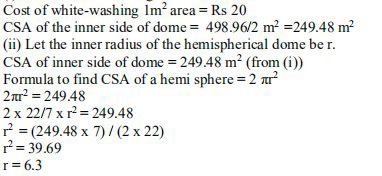# NCERT Solutions Class 9 Mathematics Solutions for Surface Areas and Volumes - Exercise 13.8 in Chapter 13 - Surface Areas and Volumes

A dome of a building is in the form of a hemi sphere. From inside, it was white-washed at the

cost of Rs. 4989.60. If the cost of white-washing is Rs 20 per square meter, find the

(i) inside surface area of the dome, (ii) volume of the air inside the dome

(Assume π = 22/7)

(i)Cost of white-washing the dome from inside = Rs 4989.60The volume of air inside the dome =Volume of hemispherical dome

Using the formula, the volume of the hemisphere = 2 / 3 \pi \mathrm{r}^{3}

= 2/3 x 22/7 x 6.3 x 6.3 x 6.3

= 523.908

= 523.9 (approx.)

Answer: Volume of air inside the dome is 523.9 \mathrm{m}^{3}

Related Questions

Lido

Courses

Teachers

Book a Demo with us

Syllabus

Maths
CBSE
Maths
ICSE
Science
CBSE

Science
ICSE
English
CBSE
English
ICSE
Coding

Terms & Policies

Selina Question Bank

Maths
Physics
Biology

Allied Question Bank

Chemistry
Connect with us on social media!